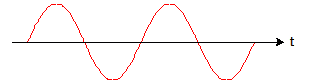# An object oscillating in simple harmonic motion

• lorenz0

#### lorenz0

Homework Statement
An object oscillates in simple harmonic motion, reaching a maximum velocity of ##1.2m/s## whenever it passes through the central position, which occurs every ##3.6s##.
Find the amplitude and maximum acceleration of the harmonic motion.
Relevant Equations
##v_{max}=\omega A##, ##a_{max}=\omega^2 A##, ##\omega=\frac{2\pi}{T}##
Since it passes through the origin every ##3.6s## the period is ##T=3.6s## hence ##\omega=\frac{2\pi}{\omega}=\frac{2\pi}{3.6}\frac{rad}{s}## thus ##A=\frac{v_{max}}{\omega}=\frac{1.2}{\frac{2\pi}{3.6}}m\simeq 0.69m## and ##a_{max}=\omega^2 A=(\frac{2\pi}{T})^2 A=(\frac{2\pi}{3.6})^2 \cdot 0.69\simeq 2.1\frac{m}{s^2}##.

What I have done makes sense to me so I don't understand why the solutions to this problem state that ##A=2.8m## and ##a_{max}=0.52\frac{m}{s^2}##. Comment are welcome, thanks.

Since it passes through the origin every ##3.6s## the period is ##T=3.6s##
It passes through the origin twice per period!

•lorenz0
Start with a sketch of a generic SHM and label the times of the zero-crossings:•lorenz0
What I have done makes sense to me so I don't understand why the solutions to this problem state that ##A=2.8m## and ##a_{max}=0.52\frac{m}{s^2}##. Comment are welcome, thanks.
These don't look right to me. Especially the acceleration, which looks very low.

What I have done makes sense to me so I don't understand why the solutions to this problem state that ##A=2.8m## and ##a_{max}=0.52\frac{m}{s^2}##. Comment are welcome, thanks.
To get those answers you need the period to be ##14.4s##.

It passes through the origin twice per period!
Ah, I understand my mistake now, thanks!

Homework Statement:: An object oscillates in simple harmonic motion, reaching a maximum velocity of ##1.2m/s## whenever it passes through the central position, which occurs every ##3.6s##.
That's a poorly worded statement. The oscillator will pass through the central position twice per period, but will have a maximum velocity only once per period. The statement can be fixed by specifying that it reaches a maximum speed of 1.2 m/s whenever it passes through the central position.